GFG App
Open AppBrowser
Continue

# Construct DFA with Σ = {0, 1} and Accept All String of Length At Most 2

Construct a DFA for a language accepting strings of length at most two, over input alphabets Σ = {0,1}. So that means in DFA, language consisting of a string of lengths 0, 1, and 2 is present. Length of string zero means when the machine doesn’t get any symbol as an input but it still accepts something(epsilon).

### Approach:

1. First, try to make the language with the help of string conditions.
2. Find the minimum possible string that satisfies the condition of length at most two.
3. So, the required strings which have lengths 0, 1, and 2 will be the strings in the given language.

### Concept:

String with length 0: String with length zero also satisfies our condition. To denote the string with length zero we use epsilon(ε).

`L1 = {ε}`

String with length 1: String with length 1, we have two strings “a” and “b” because we have an input alphabet a and b only.

`L2 = {a, b}`

String with length 2: String with length 2, we have four strings that satisfy our needs “aa”, “ab”, “ba”, and “bb”.

`L2 = {aa, ab, ba, bb}`

So all these strings satisfy the condition of the string of length at most two. So the language generated by these strings consists of these all strings. This is shown below:

`L = {ε, a, b, aa, ab, ba, bb}`

So here the approach is, for satisfying the above strings:

### Accepting epsilon:

Design an initial state which will not take any input alphabet but it should accept the string which means it should take the string which has a length of zero. So for accepting such a string, we will make the final state to an initial state so whatever the input will come on that state it will accept it.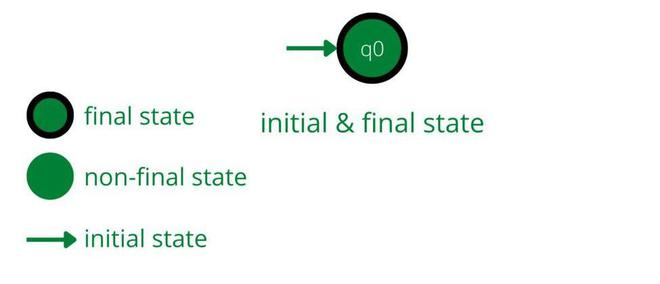### Accepting a and b:

For a and b, the transition from the initial state to the new state which is q1 while it will take the transition from the initial to q1 state it should be accepted by DFA Machine so for that it should have the q1 state as a final state. If it has a self-loop for a and b on the initial state which is q0, then string length may be increased but the constraint is it should have a fixed length which is 0, 1, and 2.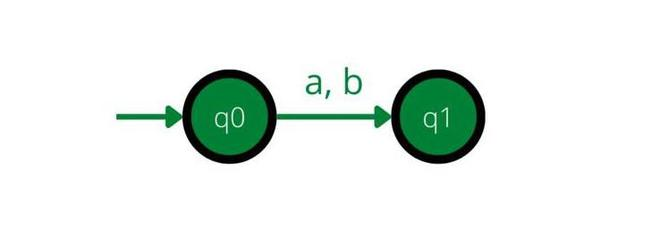### Accepting aa, ab, ba, and bb:

For this set of strings, the new state will be required. So the new state is named q2 and the transition from q1 to q2 is required. The state q2 must be the final state as it should accept the given strings satisfied by the given condition.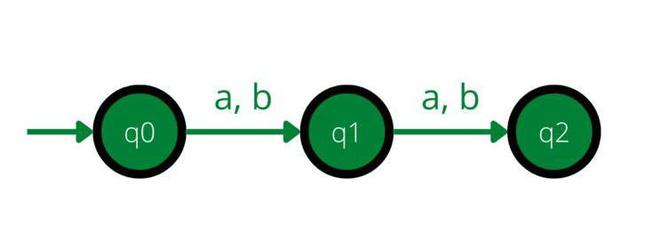### Reject all other strings:

The transition of two input symbols for q0, and q1 is done but not for q2. When the machine state is q2 whatever the input string will come, the length of the string will be increased but it should not happen to a given DFA machine, it must not take the string of length greater than 2. So here machine needs the one trap/dead state so it will help to discard the strings which have a length greater than 2.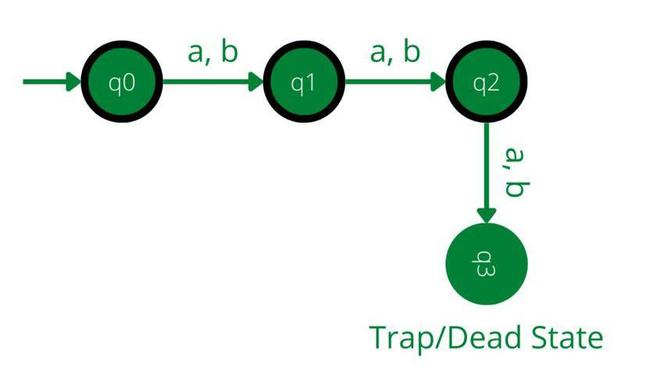### Trap State:

In the trap state, it doesn’t matter which input alphabet will come because the length of the string is already increased. So it will discard all strings no matter what input alphabet is there.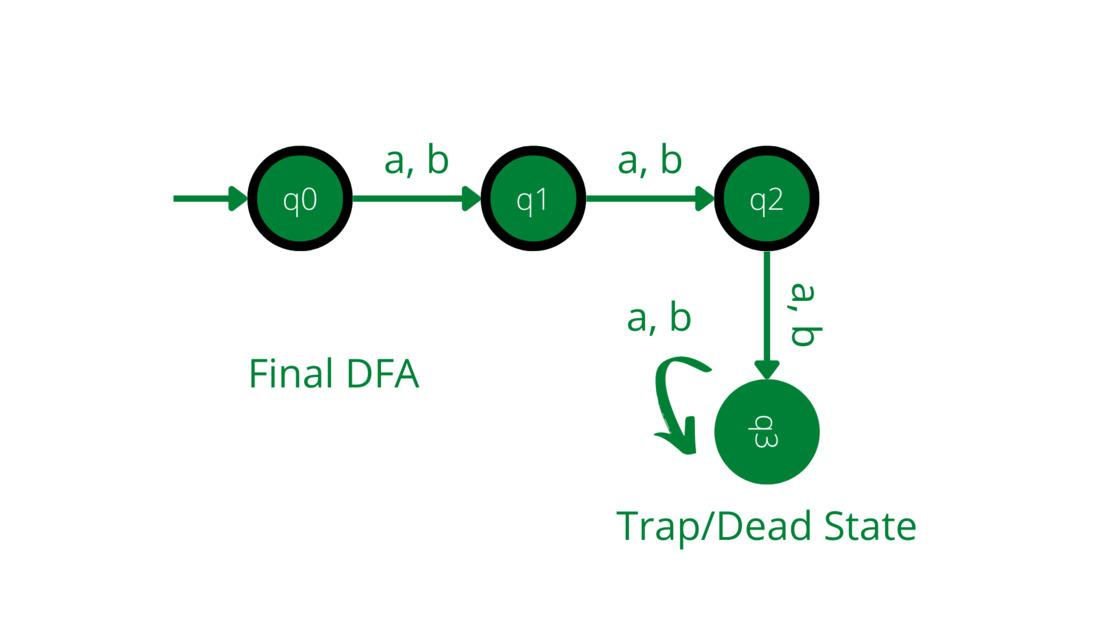My Personal Notes arrow_drop_up